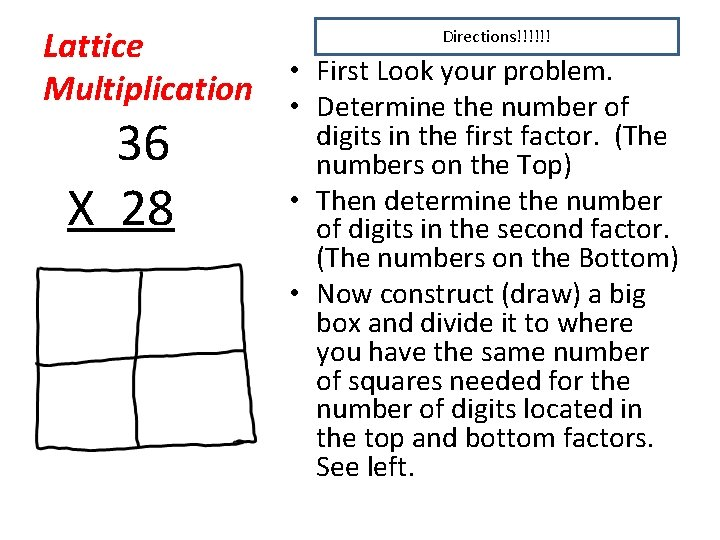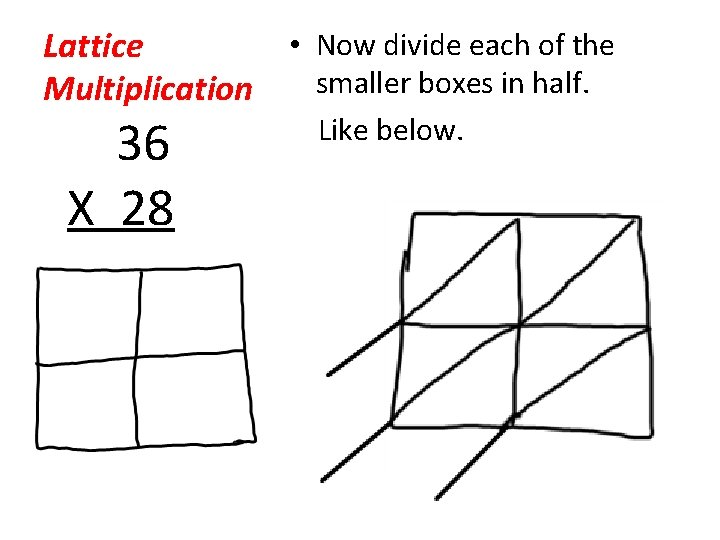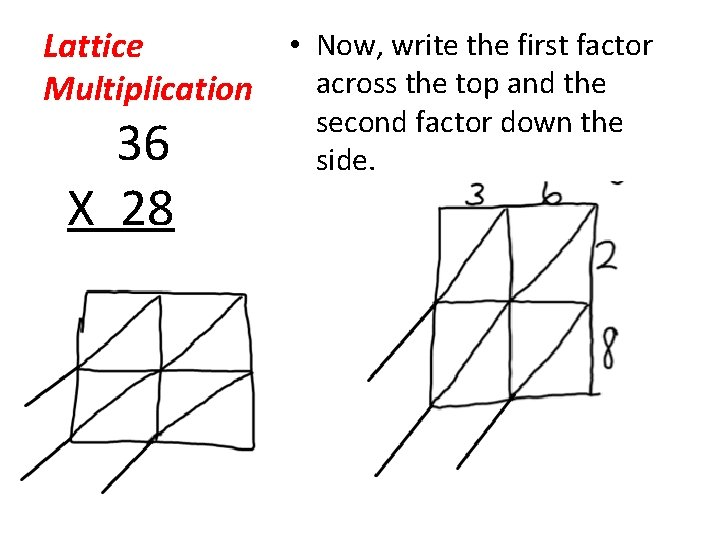# Lattice Multiplication A NEW way to Multiply By

• Slides: 7Lattice Multiplication A NEW way to Multiply By: Mrs. Overley 4 th Grade Math TeacherLattice • First Look your problem. Multiplication • Determine the number of Directions!!!!!! 36 X 28 digits in the first factor. (The numbers on the Top) • Then determine the number of digits in the second factor. (The numbers on the Bottom) • Now construct (draw) a big box and divide it to where you have the same number of squares needed for the number of digits located in the top and bottom factors. See left.• Now divide each of the Lattice smaller boxes in half. Multiplication 36 X 28 Like below.• Now, write the first factor Lattice across the top and the Multiplication 36 X 28 second factor down the side.Lattice Multiplication 36 X 28 • Now begin to multiply the first factors together, the tens digit goes in the top half of the box and the ones digit goes in the bottom half.• Now continue multiplying. Lattice (If the answer is a single Multiplication digit, such as 6, then place a 0 in the top half the box and write a 6 in the bottom half. ) 36 X 28Lattice NMultiplication • Now add each of the columns diagonally. Regroup when necessary. 36 X 28 • Follow the same steps for 3 -digit by 2 -digit numbers! (Or can be used with any multiplication problem!)